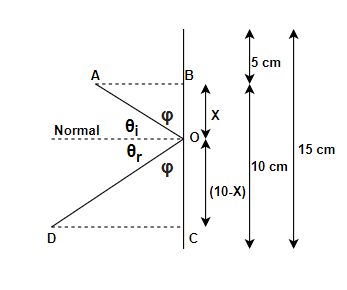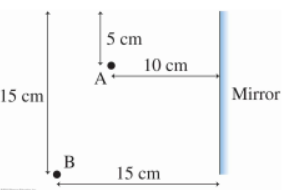# Problem: A light ray leaves point A in Figure 1, reflects from the mirror, and reaches point B. a) How far below the top edge does the ray strike the mirror? b) What if the distance from point B to the mirror was reduced by 5 cm?

###### FREE Expert SolutionThus, the angles marked with φ  are equal.

97% (451 ratings)###### Problem DetailsA light ray leaves point A in Figure 1, reflects from the mirror, and reaches point B.

a) How far below the top edge does the ray strike the mirror?

b) What if the distance from point B to the mirror was reduced by 5 cm?